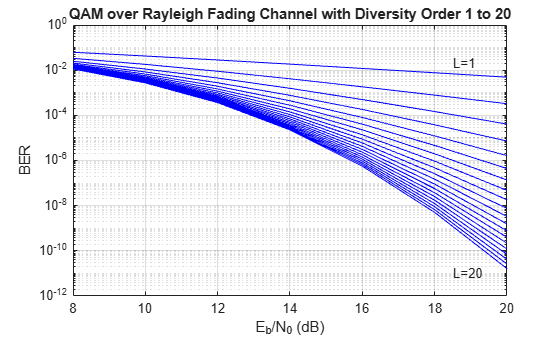Bit error rate (BER) for Rayleigh and Rician fading channels

## Syntax

```ber = berfading(EbNo,'pam',M,divorder) ber = berfading(EbNo,'qam',M,divorder) ber = berfading(EbNo,'psk',M,divorder) ber = berfading(EbNo,'depsk',M,divorder) ber = berfading(EbNo,'oqpsk',divorder) ber = berfading(EbNo,'dpsk',M,divorder) ber = berfading(EbNo,'fsk',M,divorder,coherence) ber = berfading(EbNo,'fsk',2,divorder,coherence,rho) ber = berfading(EbNo,...,K) ber = berfading(EbNo,'psk',2,1,K,phaserr) [BER,SER] = berfading(EbNo, ...) ```

## Description

### For All Syntaxes

The first input argument, `EbNo`, is the ratio of bit energy to noise power spectral density, in dB. If `EbNo` is a vector, the output `ber` is a vector of the same size, whose elements correspond to the different Eb/N0 levels.

Most syntaxes also have an `M` input that specifies the alphabet size for the modulation. `M` must have the form 2k for some positive integer `k`.

`berfading` uses expressions that assume Gray coding. If you use binary coding, the results may differ.

For cases where diversity is used, the Eb/N0 on each diversity branch is `EbNo/divorder`, where `divorder` is the diversity order (the number of diversity branches) and is a positive integer.

### For Specific Syntaxes

`ber = berfading(EbNo,'pam',M,divorder)` returns the BER for PAM over an uncoded Rayleigh fading channel with coherent demodulation.

`ber = berfading(EbNo,'qam',M,divorder)` returns the BER for QAM over an uncoded Rayleigh fading channel with coherent demodulation. The alphabet size, `M`, must be at least 4. When $k={\mathrm{log}}_{2}M$ is odd, a rectangular constellation of size $M=I×J$ is used, where $I={2}^{\frac{k-1}{2}}$ and $J={2}^{\frac{k+1}{2}}$.

`ber = berfading(EbNo,'psk',M,divorder)` returns the BER for coherently detected PSK over an uncoded Rayleigh fading channel.

`ber = berfading(EbNo,'depsk',M,divorder)` returns the BER for coherently detected PSK with differential data encoding over an uncoded Rayleigh fading channel. Only M = 2 is currently supported.

`ber = berfading(EbNo,'oqpsk',divorder)` returns the BER of coherently detected offset-QPSK over an uncoded Rayleigh fading channel.

`ber = berfading(EbNo,'dpsk',M,divorder)` returns the BER for DPSK over an uncoded Rayleigh fading channel. For DPSK, it is assumed that the fading is slow enough that two consecutive symbols are affected by the same fading coefficient.

`ber = berfading(EbNo,'fsk',M,divorder,coherence)` returns the BER for orthogonal FSK over an uncoded Rayleigh fading channel. `coherence` should be `'coherent'` for coherent detection, or `'noncoherent'` for noncoherent detection.

`ber = berfading(EbNo,'fsk',2,divorder,coherence,rho)` returns the BER for binary nonorthogonal FSK over an uncoded Rayleigh fading channel. `rho` is the complex correlation coefficient. See Nonorthogonal 2-FSK with Coherent Detection for the definition of the complex correlation coefficient and how to compute it for nonorthogonal BFSK.

`ber = berfading(EbNo,...,K)` returns the BER over an uncoded Rician fading channel, where `K` is the ratio of specular to diffuse energy in linear scale. For the case of `'fsk'`, `rho` must be specified before `K`.

`ber = berfading(EbNo,'psk',2,1,K,phaserr)` returns the BER of BPSK over an uncoded Rician fading channel with imperfect phase synchronization. `phaserr` is the standard deviation of the reference carrier phase error in radians.

`[BER,SER] = berfading(EbNo, ...)` returns both the BER and SER.

## Examples

collapse all

Generate a vector of Eb/No values to evaluate.

`EbNo = 8:2:20;`

Initialize the BER results vector.

`ber = zeros(length(EbNo),20);`

Generate BER vs. Eb/No curves for 16-QAM in a fading channel. Vary the diversity order from 1 to 20.

```for L = 1:20 ber(:,L) = berfading(EbNo,'qam',16,L); end```

Plot the results.

```semilogy(EbNo,ber,'b') text(18.5, 0.02, sprintf('L=%d',1)) text(18.5, 1e-11, sprintf('L=%d',20)) title('QAM over fading channel with diversity order 1 to 20') xlabel('E_b/N_0 (dB)') ylabel('BER') grid on```## Limitations

The numerical accuracy of this function's output is limited by approximations related to the numerical implementation of the expressions

You can generally rely on the first couple of significant digits of the function's output.

## Alternatives

As an alternative to the `berfading` function, invoke the BERTool GUI (`bertool`), and use the Theoretical tab.

## References

 Proakis, John G., Digital Communications, 4th ed., New York, McGraw-Hill, 2001.

 Modestino, James W., and Mui, Shou Y., Convolutional code performance in the Rician fading channel, IEEE Trans. Commun., 1976.

 Cho, K., and Yoon, D., “On the general BER expression of one- and two-dimensional amplitude modulations”, IEEE Trans. Commun., Vol. 50, Number 7, pp. 1074-1080, 2002.

 Lee, P. J., “Computation of the bit error rate of coherent M-ary PSK with Gray code bit mapping”, IEEE Trans. Commun., Vol. COM-34, Number 5, pp. 488-491, 1986.

 Lindsey, W. C., “Error probabilities for Rician fading multichannel reception of binary and N-ary signals”, IEEE Trans. Inform. Theory, Vol. IT-10, pp. 339-350, 1964.

 Simon, M. K , Hinedi, S. M., and Lindsey, W. C., Digital Communication Techniques – Signal Design and Detection, Prentice-Hall, 1995.

 Simon, M. K., and Alouini, M. S., Digital Communication over Fading Channels – A Unified Approach to Performance Analysis, 1st ed., Wiley, 2000.

 Simon, M. K , “On the bit-error probability of differentially encoded QPSK and offset QPSK in the presence of carrier synchronization”, IEEE Trans. Commun., Vol. 54, pp. 806-812, 2006.